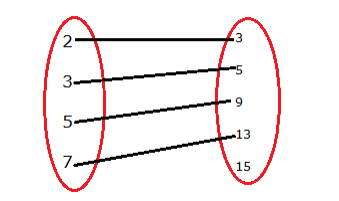# Let A = {2, 3, 5, 7} and B = {3, 5, 9, 13, 15}.

Question:

Let $A=\{2,3,5,7\}$ and $B=\{3,5,9,13,15\} .$ Let $f=\{(x, y): x \in A, y \in B$ and $y=$2 x-1\}$Write f in roster form. Show that f is a function from A to B. Find the domain and range of f. Solution: Given: A = {2, 3, 5, 7} and B = {3, 5, 9, 13, 15}$f=\{(x, y): x \in A, y \in B$and$y=2 x-1\}$For$x=2y=2 x-1y=2(2)-1y=3 \in B$For$x=3y=2 x-1y=2(3)-1y=5 \in B$For$x=5y=2 x-1y=2(5)-1y=9 \in B$For$x=7y=2 x-1y=2(7)-1\mathrm{y}=13 \in \mathrm{B}\therefore f=\{(2,3),(3,5),(5,9),(7,13)\}$Now, we have to show that f is a function from A to B Function: (i) all elements of the first set are associated with the elements of the second set. (ii) An element of the first set has a unique image in the second set.$f=\{(2,3),(3,5),(5,9),(7,13)\}$Here, (i) all elements of set A are associated with an element in set B. (ii) an element of set$\mathrm{A}$is associated with a unique element in set$\mathrm{B}\$.

∴ f is a function.

Dom (f) = 2, 3, 5, 7

Range (f) = 3, 5, 9, 13